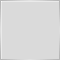# Something with iMAOnArray1140

Hi all,

I'm on a verge of bilding multi pairs EA and I found some problem.

I've try to trace the problem and checking some codes but I'm, stuck on this.

Is anybody know why ma1 and ma2 are resulting different?

```   int MAPeriod=100;
int MAMethod=0;

double clos;
for(int k=0;k<500;k++)
{
clos[k]=Close[k];
}

double ma1=iMAOnArray(clos,0,MAPeriod,0,MAMethod,1);
double ma2=iMA(Symbol(),0,MAPeriod,0,MAMethod,PRICE_CLOSE,1);```Moderator
2317

devilian1899:
Hi all,

I'm on a verge of bilding multi pairs EA and I found some problem.

I've try to trace the problem and checking some codes but I'm, stuck on this.

Is anybody know why ma1 and ma2 are resulting different?

```   int MAPeriod=100;
int MAMethod=0;

double clos;
ArraySetAsSeries(clos,true);

for(int k=0;k<500;k++)
{
clos[k]=Close[k];
}

double ma1=iMAOnArray(clos,0,MAPeriod,0,MAMethod,1);
double ma2=iMA(Symbol(),0,MAPeriod,0,MAMethod,PRICE_CLOSE,1);```27833

Without the array being AsSeries, you are asking for the MA(1) so the MAoa only has clos and clos to work with.1140

Oh thank you.. forgot to add that part.
honest_knave:

I see, thanks.

WHRoeder:
Without the array being AsSeries, you are asking for the MA(1) so the MAoa only has clos and clos to work with.